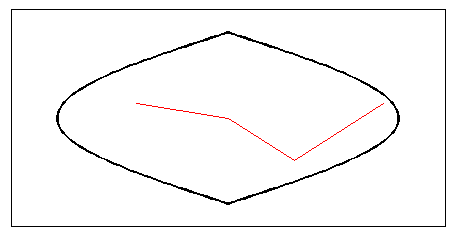# linem

Project line object on map axes

## Syntax

```h = linem(lat,lon) h = linem(lat,lon,linetype) h = linem(lat,lon,PropertyName,PropertyValue,...) h = linem(lat,lon,z) ```

## Description

`h = linem(lat,lon)` displays projected line objects on the current map axes. `lat` and `lon` are the latitude and longitude coordinates, respectively, of the line object to be projected. Note that this ordering is conceptually reversed from the MATLAB® `line` function, because the vertical (y) coordinate comes first. However, the ordering latitude, then longitude, is standard geographic usage. `lat` and `lon` must be the same size and in the `AngleUnits` of the map axes. The object handle for the displayed line can be returned in `h`.

`h = linem(lat,lon,linetype)` where `linetype` is a `linespec` that specifies the line style.

`h = linem(lat,lon,PropertyName,PropertyValue,...)` allows the specification of any number of property name/property value pairs for any properties recognized by the MATLAB `line` function except for `XData`, `YData`, and `ZData`.

`h = linem(lat,lon,z)` displays a line object in three dimensions, where `z` is the same size as `lat` and `lon` and contains the desired altitude data. `z` is independent of `AngleUnits`. If omitted, all points are assigned a z-value of 0 by default.

The units of `z` are arbitrary, except when using the `globe` projection. In the case of `globe`, `z` should have the same units as the radius of the earth or semimajor axis specified in the `'geoid'` (reference ellipsoid) property of the map axes. This implies that when the reference ellipsoid is a unit sphere, the units of `z` are earth radii.

`linem` is the mapping equivalent of the MATLAB `line` function. It is a low-level graphics function for displaying line objects in map projections. Ordinarily, it is not used directly. Use `plotm` or `plot3m` instead.

## Examples

```axesm sinusoid; framem linem([15; 0; -45; 15],[-100; 0; 100; 170],'r-')```